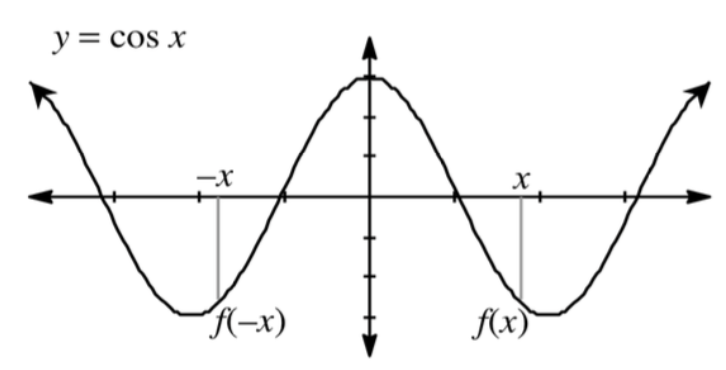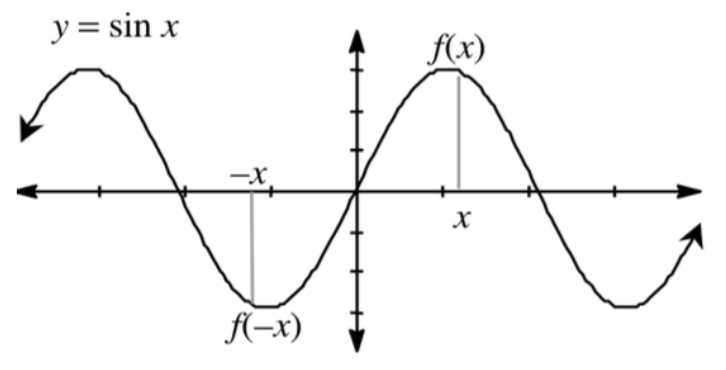### Home > PC > Chapter 7 > Lesson 7.1.2 > Problem7-24

7-24.

The sine and cosine functions can be classified as even, odd, or neither. The diagrams below show why.

1. This shows that $\cos\left(−x\right) =$ ____ and $\sin\left(−x\right) =$ ____.

$\cos\left(−x\right) = \cos\left(x\right)$ and $\sin\left(−x\right) = −\sin x$

2. Classify the $y = \sin x$ and $y = \cos x$ as either even or odd.

Sine is odd, cosine is even.